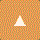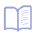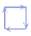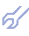As with the Combinatorial algorithm, the output variable must be specified in advance by the person in charge of modeling, which corresponds to the use of so-called explicit templates [16,25]. In each layer, the F best models are used to successively extend the input data sample. In Multilayered Iterative (MIA) recurrent algorithm the iteration rule remains unchanged from one layer to next. As it shown the first layer tests the models, that can be derived from the information contained in any two columns of the sample. The second layer uses information from four columns; the third, from any eight columns, etc. The exhaustive-search termination rule is the same as for the Combinatorial algorithm: in each layer the optimal models are selected by the minimum of external criterion.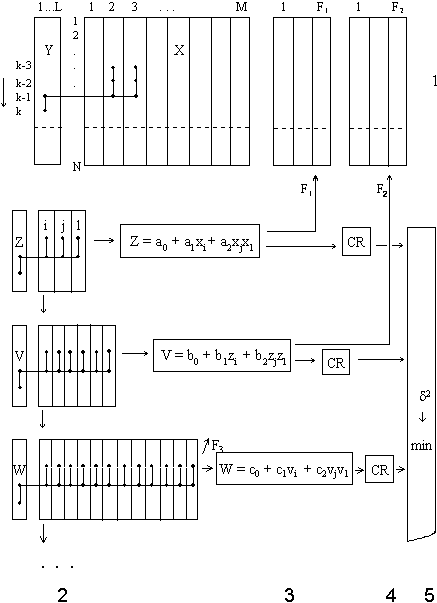Output model: Yk+1 = d0 + d1x1k + d2 x2k+ ... +dm xM k xM-1 k where:          1 - data sampling;          2 - layers of partial descriptions complexing;          3 - form of partial descriptions;          4 - choice of optimal models;          5 - additional model definition by discriminating criterion;          F1 and F2 - number of variables for data sampling extension. MIA should be used when it is needed to handle a big number of variables (up to 500). This algorithm also can be modified in such way that at each layer a set of F best variables is selected and at next layer only this variables are used. MIA may contain in some cases the "multilayerness error" when effective variable are not selected which is analogical to statistical error of control systems. Multilayered GMDH algorithms can be used for solving of incorrect and ill-defined modeling problems, i.e. in the cases when number of observations is less than variables N < M. The regression analysis methods are inapplicable in this case, because they give not possibility to build the only model, which is adequate to process in this case. Originally GMDH was proposed as addition to regression analysis of two procedures: 1) for sets of model candidates generation: different algorithms mainly differs one from another by the way of models candidates sets generation; 2) for search of optimal model by external criterion. Recent time two additional procedures are added: 3) preliminary handling of data sample by clusterization algorithm. Initial data sample should be changed to the set of cluster centers coordinates. 4) models received are used as active neurons in twice-multilayered neuronet for additional increase of modeling accuracy.